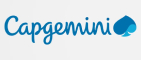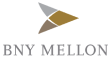New update is available. Click here to update.
Topics

# Find Duplicate in Array

Easy0/40
Average time to solve is 15m+7 more companies

## Problem statement

You are given an array of integers 'ARR' containing N elements. Each integer is in the range [1, N-1], with exactly one element repeated in the array.

Your task is to find the duplicate element. The duplicate element may be repeated more than twice in the error, but there will be exactly one element that is repeated in the array.

Note :

``````All the integers in the array appear only once except for precisely one integer which appears two or more times.
``````
Detailed explanation ( Input/output format, Notes, Images )
Constraints :
``````1 <= T <= 5
1 <= N <= 10^5
1 <= ARR[i] <= N - 1

Time Limit: 1 sec
``````

#### Sample Input 1:

``````1
3
1 1 2
``````

#### Sample Output 1:

``````1
``````

#### Explanation of Sample Input 1:

``````1 is repeated in the array, hence function returns 1.
``````

#### Sample Input 2:

``````3
5
1 3 4 2 2
5
3 1 3 4 2
3
1 1 1
``````

#### Sample Output 2:

``````2
3
1
``````Console